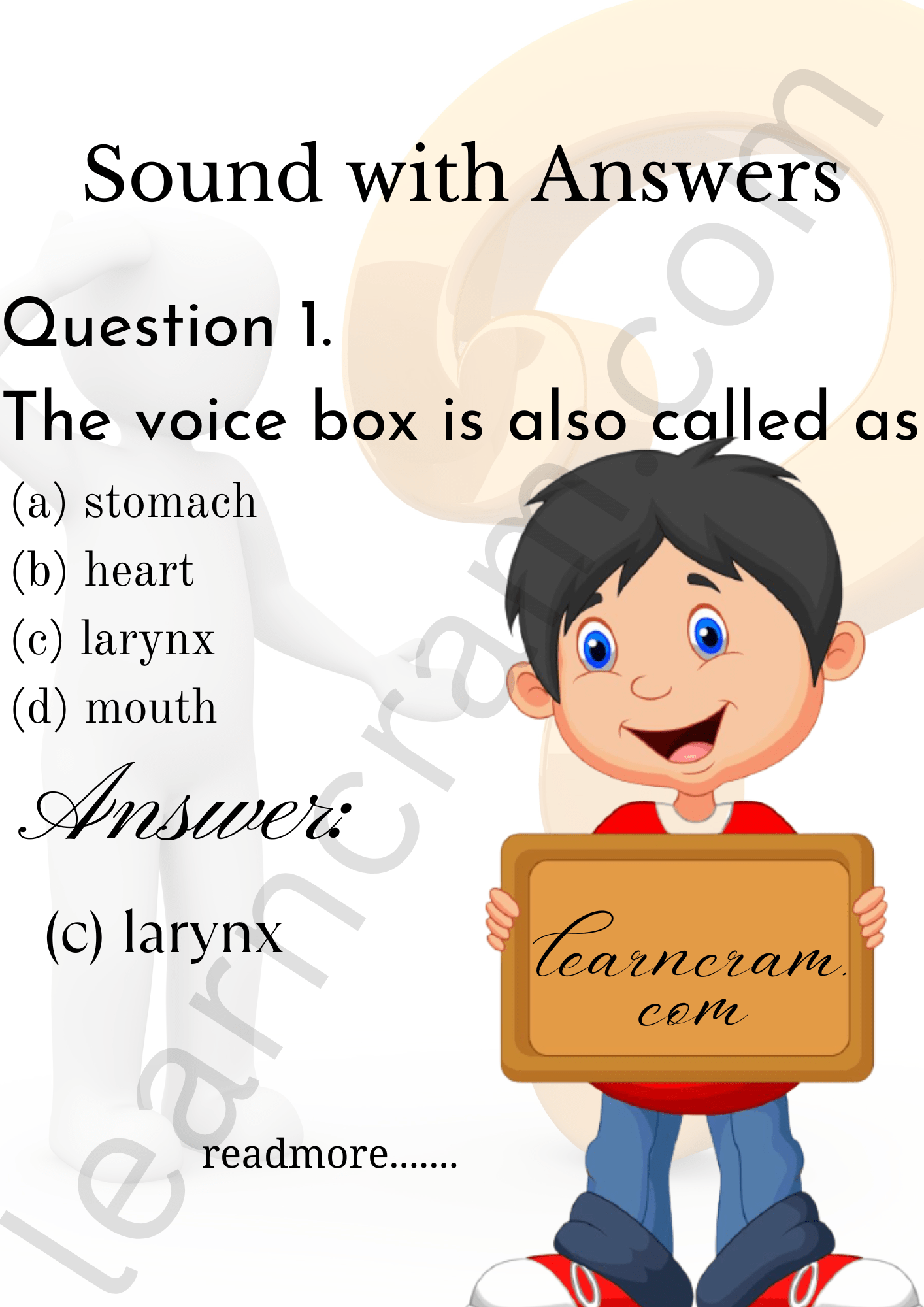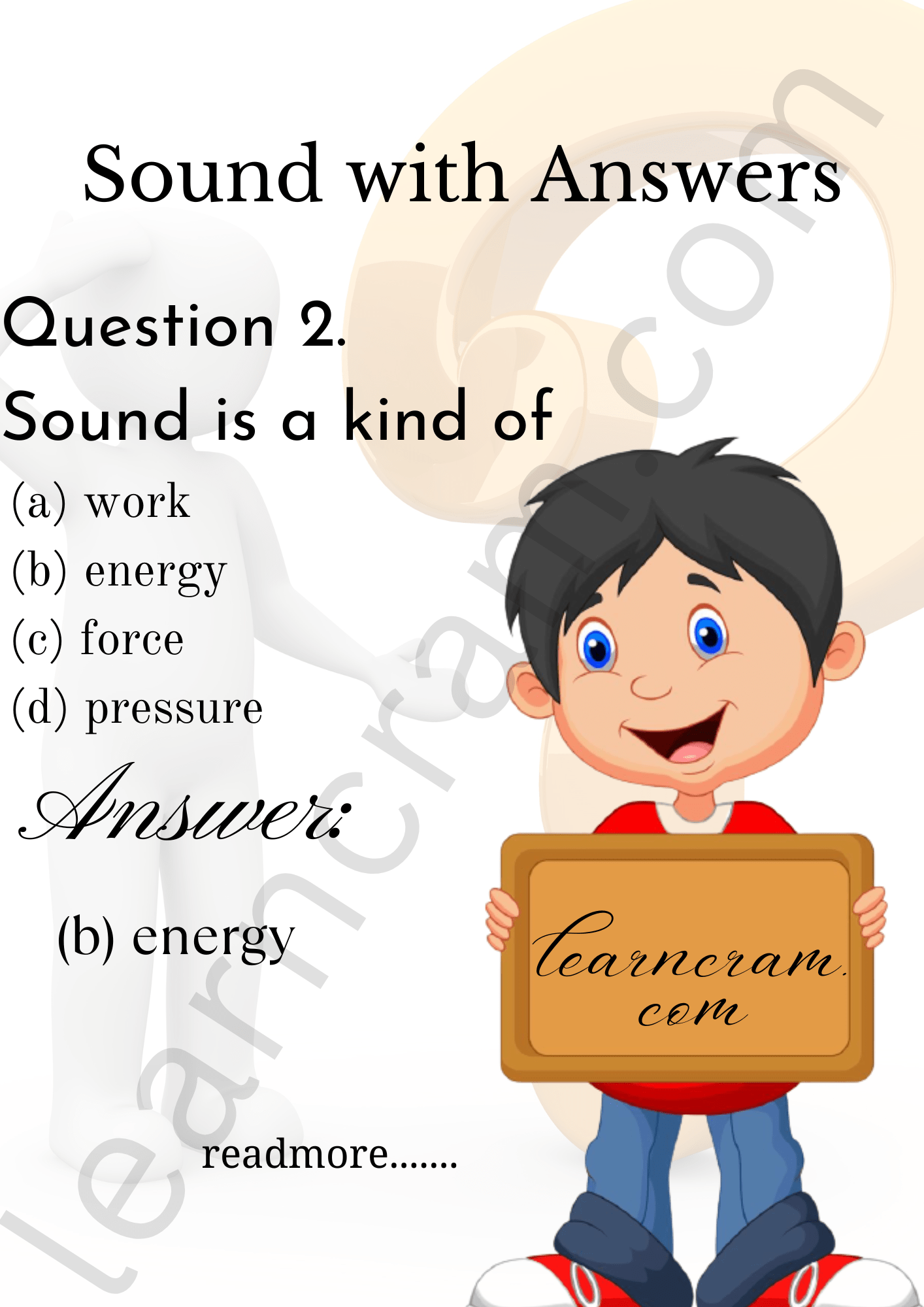# MCQ Questions for Class 8 Science Chapter 13 Sound with Answers

We have compiled the NCERT MCQ Questions for Class 8 Science Chapter 13 Sound with Answers Pdf free download covering the entire syllabus. Practice MCQ Questions for Class 8 Science with Answers on a daily basis and score well in exams. Refer to the Sound Class 8 MCQs Questions with Answers here along with a detailed explanation.

## Sound Class 8 MCQs Questions with Answers

Choose the correct option.

Question 1.
The voice box is also called as
(a) stomach
(b) heart
(c) larynx
(d) mouthQuestion 2.
Sound is a kind of
(a) work
(b) energy
(c) force
(d) pressureQuestion 3.
The hearing range of human ear is
(a) 20 Hz to 20,000 Hz
(b) less than 20 Hz
(c) more than 20,000 Hz
(d) 20 Hz to 25,000 Hz

Answer: (a) 20 Hz to 20,000 Hz

Question 4.
Pitch of sound is determined by its
(a) frequency
(b) speed
(c) amplitude
(d) loudness

Question 5.
The frequency of subsonic sound is
(a) more than 20 Hz
(b) 100 Hz
(c) less than 20 Hz
(d) more than 20,000 Hz

Answer: (c) less than 20 Hz

Question 6.
Cochlea is a part of
(a) hearing organ
(b) sound producing organ
(c) muscular organ
(d) air pollution

Question 7.
1 hertz is equal to
(a) 1 vibration per minute
(b) 10 vibrations per minute
(c) 60 vibrations per minute
(d) 600 vibrations per minute

Answer: (c) 60 vibrations per minute

Question 8.
Sound cannot travel through
(a) air
(b) water
(c) iron
(d) vacuum

Question 9.
The sound in the audible range is called
(a) ultrasonic sound
(b) sonic sound
(c) subsonic sound
(d) light sound

Question 10.
Speed is
(a) $$\frac {Distance travelled}{Time}$$
(b) $$\frac {Time}{Distance travelled}$$
(c) Distance travelled × Time
(d) Time + Distance travelled

Answer: (a) $$\frac {Distance travelled}{Time}$$

Question 11.
A pendulum oscillates 20 times in 4 seconds. Find its time period.
(a) 0.05 sec.
(b) 0.001 sec.
(c) 0.2 sec.
(d) 0.1 sec.

Question 12.
Loudness of sound is determined by
(a) pitch
(b) frequency
(c) amplitude
(d) time period

Question 13.
The number of vibrations made by a vibrating body in one second is
(a) frequency
(b) noise
(c) loudness
(d) pitch

Question 14.
The maximum displacement of a body from its mean position is called
(a) amplitude
(b) oscillation
(c) periodic motion
(d) frequency

Question 15.
The velocity of sound at 20°C is approximately
(a) 3400 m/sec.
(b) 340 m/sec.
(c) 430 m/sec.
(d) 304 m/sec.

Question 16.
Sound usually travels through which medium to reach our ears?
(a) Air
(b) Water
(c) Wood
(d) Asbestos

Question 17.
What is the speed of sound in air?
(a) 110 m/s
(b) 220 m/s
(c) 330 m/s
(d) 440 m/s

Question 18.
What is the length of vocal cords in men?
(a) 5 mm
(b) 10 mm
(c) 15 mm
(d) 20 mm

Question 19.
What is the length of vocal cords in women?
(a) 5 mm
(b) 10 mm
(c) 15 mm
(d) 20 mm

Question 20.
A vibrating body should oscillate minimum how many times per second to make a sound audible for humans?
(a) 10 times
(b) 20 times
(c) 30 times
(d) 40 times

Question 21.
The voice box is also called as
(a) pharynx
(b) throat
(c) larynx
(d) windpipe

Question 22.
Speed of sound in solids is the speed of sound in liquids.
(a) same as
(b) greater than
(c) less than
(d) both (b) and (c)

Question 23.
To and fro or back and forth motion of an object is termed as
(a) frequency
(b) amplitude
(c) vibration
(d) time period

Question 24.
The sound above ……………… is physically painful.
(a) 20 dB
(b) 40 dB
(c) 60 dB
(d) 80 dB

Question 25.
If we tighten the strings of an instrument, pitch will be
(a) higher
(b) lower
(c) constant
(d) no pitch

Question 26.
In dholak, sound is produced due to
(a) stretched membrane
(b) stretched strings
(c) air column
(d) none of these

Question 27.
The human ear can hear sounds having frequency in range from
(a) 200 to 20,000 Hz
(b) 20 to 2000 Hz
(c) 200 to 2000 Hz
(d) 20 to 20,000 Hz

Answer: (d) 20 to 20,000 Hz

Question 28.
Sound having frequency below 20 Hz is called
(a) infrasound
(b) ultrasound
(c) megasound
(d) microsound

Fill in the Blanks

Question 1.
In human beings sound is produced by ………………..

Question 2.
Sound of frequency lower than 20 Hz is called the ………………..

Question 3.
The ……………….. nerve is also present in the inner ear.

Question 4.
Sounds which are unpleasant to the ear is called ………………..

Question 5.
Too much noise in our surroundings that causes discomfort is called ………………..

Question 6.
The speed of sound is maximum in ………………..

Question 7.
Sounds of frequencies higher than 20,000 Hz are called the ……………….. sound.

Question 8.
……………….. is the time taken by a vibrating body for one complete vibration.

Question 9.
Above ……………….. the noise becomes physically painful.

Question 10.
Plantation on the roadside can reduce ………………..

Question 11.
The hearing range of human ears is ………………..

Answer: 20 Hz to 20,000 Hz

Question 12.
The loudness of normal breathing of human is ………………..

Question 13.
Vibration is a repeated ……………….. and ……………….. motion.

Question 14.
The loudness of sound is determined by the ……………….. of vibration.

Question 15.
The human voice box is called ………………..

Question 16.
Sound absorbing materials are required in an auditorium to avoid …………………….

Question 17.
The two kinds of sounds we cannot hear are ……………………. and …………………….

Question 18.
……………………. of any kind is the principle source of production of sound.

Question 19.
…………………… is an instrument used in the laboratory to produce sound of a fixed frequency.

Question 20.
The vibrations of a simple pendulum are also known as …………………….

Question 21.
A soft board will ……………………. most of the sound falling on it.

True or False

Question 1.
All human beings can hear sounds of frequencies upto 60,000 Hz.

Question 2.
The sound in a sitar is produced by plucking its strings.

Question 3.
Sound cannot travel through vacuum.

Question 4.
Sound does not need a medium for its propagation.

Question 5.
The loudness is expressed in a unit called decibel.

Question 6.
Loud sounds have high frequencies.

Question 7.
Sound travel faster in air, slower in iron.

Question 8.
Light travels much faster than sound.

Question 9.
Man cannot hear sound of bats.

Question 10.
The time taken to complete one oscillation is called frequency.

Question 11.
Shriller sound has more frequency.

Question 12.
Tabla is a musical instrument.

Question 13.
The sound could not travel in solid.

Question 14.
The pitch of a sound depends on the frequency of the waves.

Question 15.
The pitch of sound produced in a guitar can be altered by reducing the vibrating length of the wire.

Question 16.
Voice box is situated at the lower end of the windpipe.

Question 17.
Vocal cords are of the same length in both men and women.

Question 18.
Sound does not need a medium for propagation.

Question 19.
The sound of sitar is produced by plucking its strings.

Question 20.
Amplitude measures the pitch of sound.

Question 21.
The SI unit of frequency is Hertz.

Match the following

 Column I Column II 1. Audible frequencies (a) 10 dB 2. Length of vocal cords in man (b) 30 dB 3. Ultrasound (c) 20 mm long 4. Normal breathing (d) 20 to 20,000 Hz 5. Soft whisper (At 5 m) (e) Hertz 6. Frequency (f) Percussion instrument 7. Unpleasant sound (g) Music 8. Pitch (h) Produced by bats 9. Tabla (i) Noise 10. Pleasant sound (j) Higher frequency